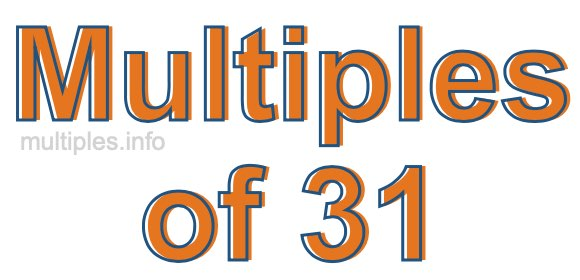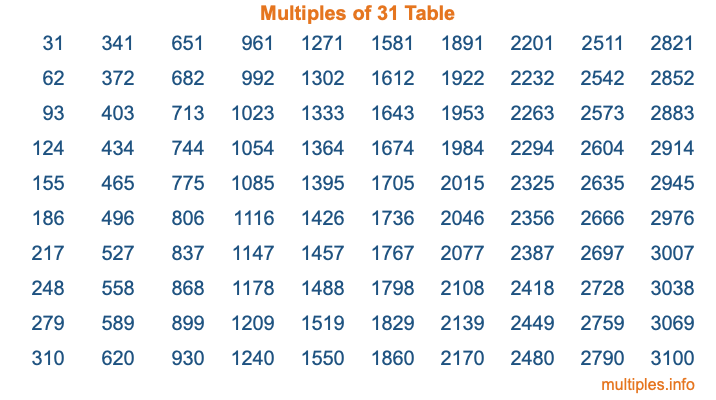Multiples of 31Welcome to the Multiples of 31 page. Here we will first teach you everything you will ever need to know about the multiples of 31, and then give you a study guide summary of everything we taught you to make sure you remember it all. Use this page to look up facts and learn information about the multiples of 31. This page will make you a multiples of thirty-one expert!

Definition of Multiples of 31
Multiples of 31 are all the numbers that when divided by 31 equal an integer. Each of the multiples of 31 are called a multiple. A multiple of 31 is created by multiplying 31 by an integer.

Therefore, to create a list of multiples of 31, you start with 1 multiplied by 31, then 2 multiplied by 31, then 3 multiplied by 31, and so on for as long as you want. Thus, the list of the first five multiples of 31 is 31, 62, 93, 124, and 155. To see a larger list of multiples of 31, see the printable image of Multiples of 31 further down on this page. We also have a category where you can choose any nth multiple of 31.

Multiples of 31 Checker
The Multiples of 31 Checker below checks to see if any number of your choice is a multiple of 31. In other words, it checks to see if there is any number (integer) that when multiplied by 31 will equal your number. To do that, we divide your number by 31. If the the quotient is an integer, then your number is a multiple of 31.

Is  a multiple of 31?

Least Common Multiple of 31 and ...
A Least Common Multiple (LCM) is the lowest multiple that two or more numbers have in common. This is also called the smallest common multiple or lowest common multiple and is useful to know when you are adding our subtracting fractions. Enter one or more numbers below (31 is already entered) to find the LCM.

Check out our LCM Calculator if you need more details about the Least Common Multiple or if you need the LCM for different numbers for adding and subtraction fractions.

nth Multiple of 31
As we stated above, 31 is the first multiple of 31, 62 is the second multiple of 31, 93 is the third multiple of 31, and so on. Enter a number below to find the nth multiple of 31.

th multiple of 31

Multiples of 31 vs Factors of 31
31 is a multiple of 31 and a factor of 31, but that is where the similarities end. All postive multiples of 31 are 31 or greater than 31. All positive factors of 31 are 31 or less than 31.

Below is the beginning list of multiples of 31 and the factors of 31 so you can compare:

Multiples of 31: 31, 62, 93, 124, 155, etc.

Factors of 31: 1, 31

As you can see, the multiples of 31 are all the numbers that you can divide by 31 to get a whole number. The factors of 31, on the other hand, are all the whole numbers that you can multiply by another whole number to get 31.

It's also interesting to note that if a number (x) is a factor of 31, then 31 will also be a multiple of that number (x).

Multiples of 31 vs Divisors of 31
The divisors of 31 are all the integers that 31 can be divided by evenly. Below is a list of the divisors of 31.

Divisors of 31: 1, 31

The interesting thing to note here is that if you take any multiple of 31 and divide it by a divisor of 31, you will see that the quotient is an integer.

Multiples of 31 Table
Below is an image of the first 100 multiples of 31 in a table. The table is in chronological order, column by column. The first column has the first ten multiples of 31, the second column has the next ten multiples of 31, and so on.The Multiples of 31 Table is also referred to as the 31 Times Table or Times Table of 31. You are welcome to print out our table for your studies.

Negative Multiples of 31
Although not often discussed or needed in math, it is worth mentioning that you can make a list of negative multiples of 31 by multiplying 31 by -1, then by -2, then by -3, and so on, to get the following list of negative multiples of 31:

-31, -62, -93, -124, -155, etc.

Multiples of 31 Summary
Below is a summary of important Multiples of 31 facts that we have discussed on this page. To retain the knowledge on this page, we recommend that you read through the summary and explain to yourself or a study partner why they hold true.

There are an infinite number of multiples of 31.

A multiple of 31 divided by 31 will equal a whole number.

31 divided by a factor of 31 equals a divisor of 31.

The nth multiple of 31 is n times 31.

The largest factor of 31 is equal to the first positive multiple of 31.

31 is a multiple of every factor of 31.

31 is a multiple of 31.

A multiple of 31 divided by a divisor of 31 equals an integer.

31 divided by a divisor of 31 equals a factor of 31.

Any integer times 31 will equal a multiple of 31.

Multiples of a Number
Here you can get the multiples of another number, all with the same attention to detail as we did for multiples of 31 on this page.

Multiples of
Multiples of 32
Did you find our page about multiples of thirty-one educational? Do you want more knowledge? Check out the multiples of the next number on our list!